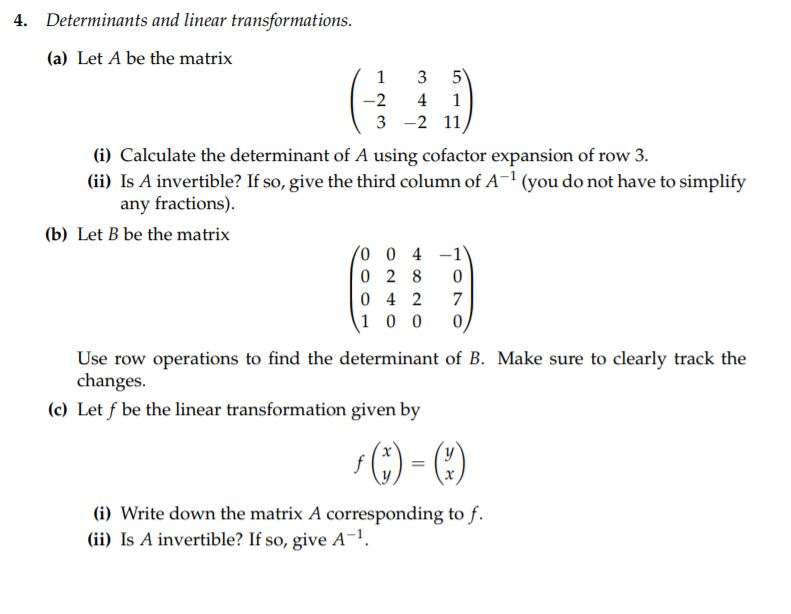# Solved: Determinants And Linear Transformations 4. (a) Let A Be The Matrix 1 -2 4 1 3 2 11 I) Calculate The Determinant Of A Using Cofactor Expansion Of Row 3. (ii) Is A Invertible? If So, Give The Th

By |Determinants and linear transformations 4. (a) Let A be the matrix 1 -2 4 1 3 2 11 i) Calculate the determinant of A using cofactor expansion of row 3. (ii) Is A invertible? If so, give the third column of A1 (you do not have to simplify any fractions) (b) Let B be the matrix 0 0 4 0 2 8 0 4 2 1 0 0 0 7 Use row operations to find the determinant of B. Make sure to clearly track the changes. (c) Let f be the linear transformation given by (i) Write down the matrix A corresponding to f (ii) Is A invertible? If so, give A